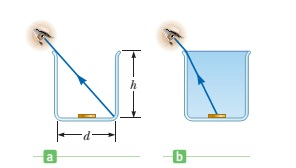# I find money in the jarA person looking into an empty container is able to see the far edge of the container’s bottom. The height of the container is $h$, and its width is $d$. When the container is completely filled with a fluid of index of refraction $n$ and viewed from the same angle, the person can see the center of a coin at the middle of the container’s bottom.

Question is that if the the container has a width of $8 \,\text{cm}$ and is filled with water, then find the height of the container in $\text{cm}$ to 2 decimal places.

Details and assumptions

• The refractive index, $n$ for water is $\dfrac{4}{3}$.
• Use calculator if required.
×

Problem Loading...

Note Loading...

Set Loading...